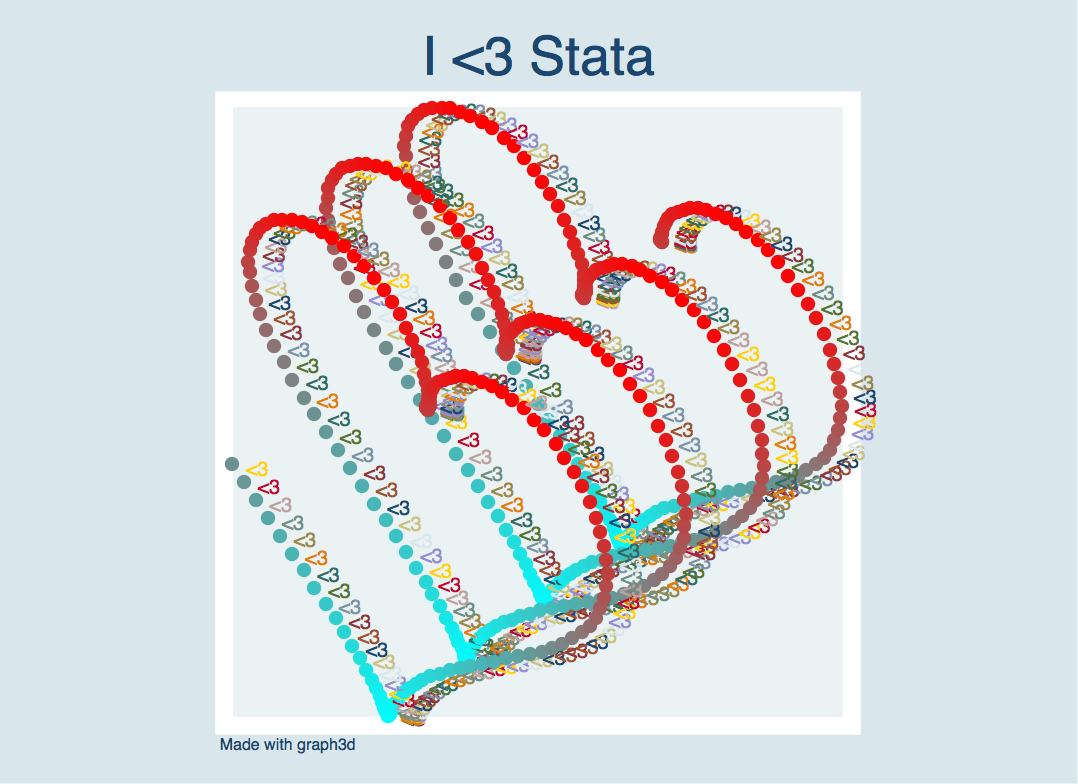GRAPH3D: Stata module to draw colored, scalable, rotatable 3D plots by Robin Jessen and Davud Rostam-Afschar

graph3d

# graph3d for Stata

. ssc install graph3d in Stata or click

Before running any of the examples, set up the exemplary dataset by running

. clear
. set obs 630
. gen x = int((_n - mod(_n-1,30) -1 ) /30 )
. gen z = mod(_n-1,30)
. gen y = normalden(x,10,3)*normalden(z,15,5)*10000
. gen w = x*z

### What Stata experts say:### Example 1: Default 3D-Plot

. graph3d x y z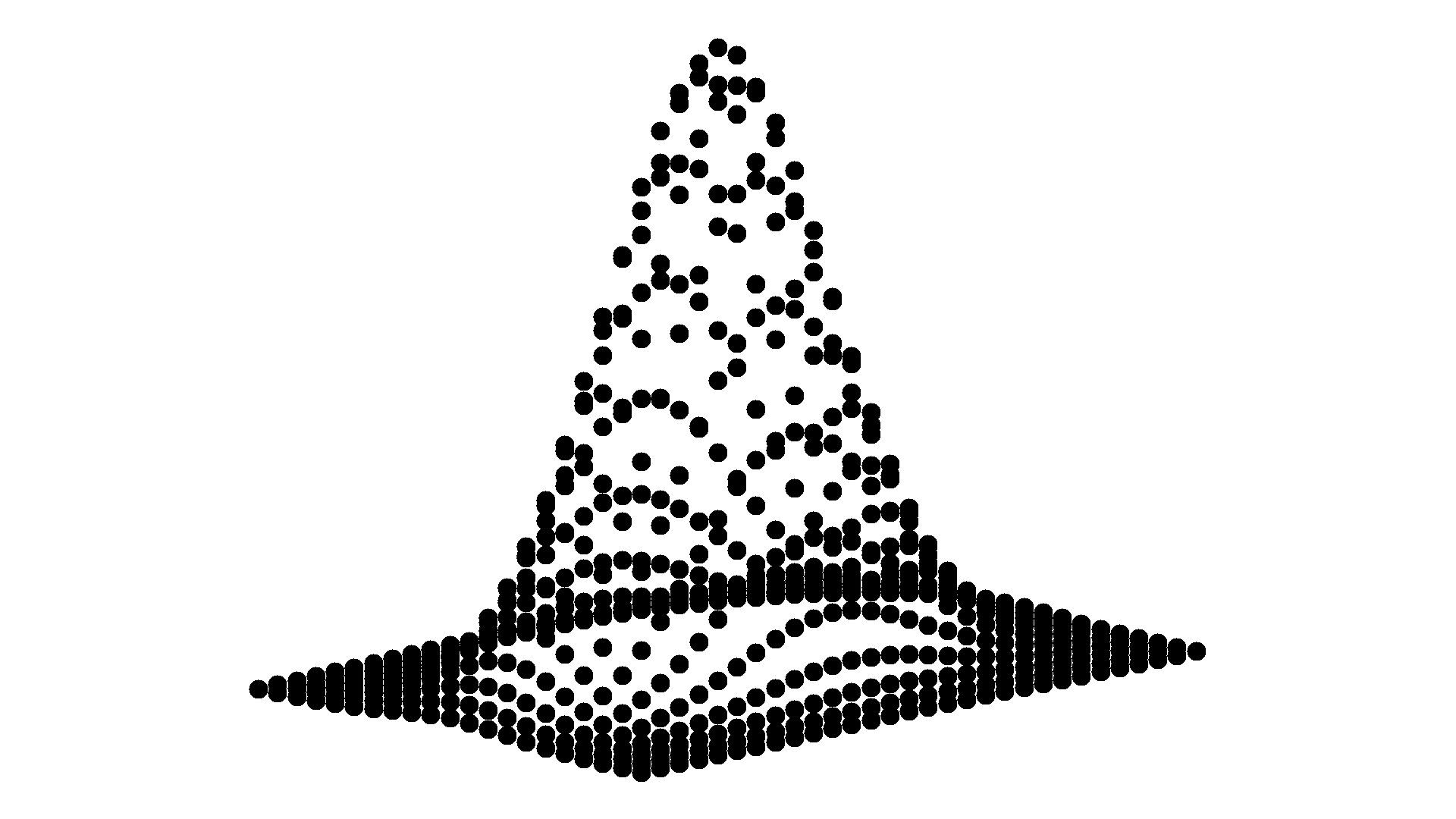### Example 2: Default 3D-Plot rotated by 75 degree about the width-axis

. graph3d x y z, xang(75)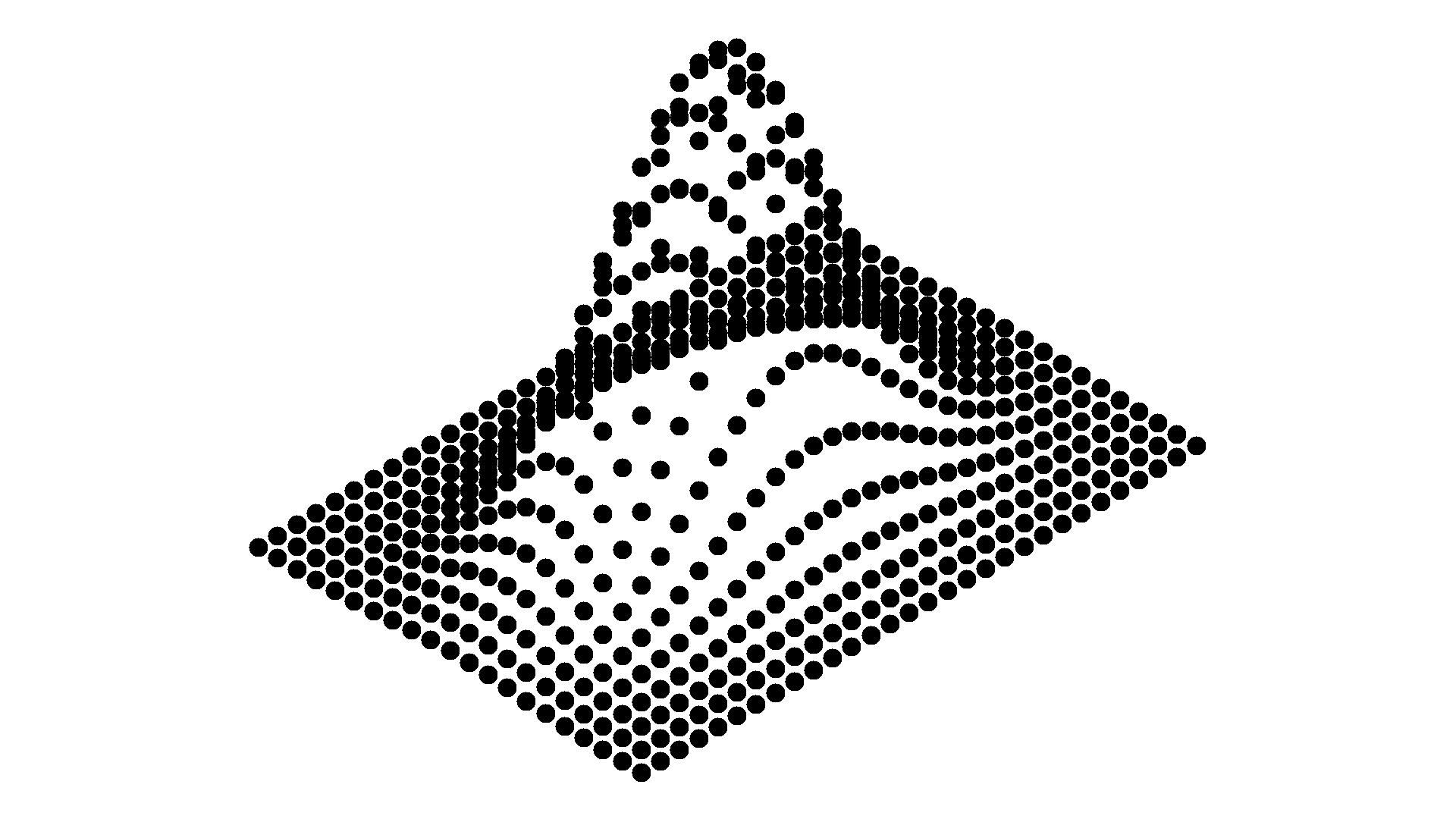### Example 3: Wireframe default 3D-Plot

. graph3d x y z, wire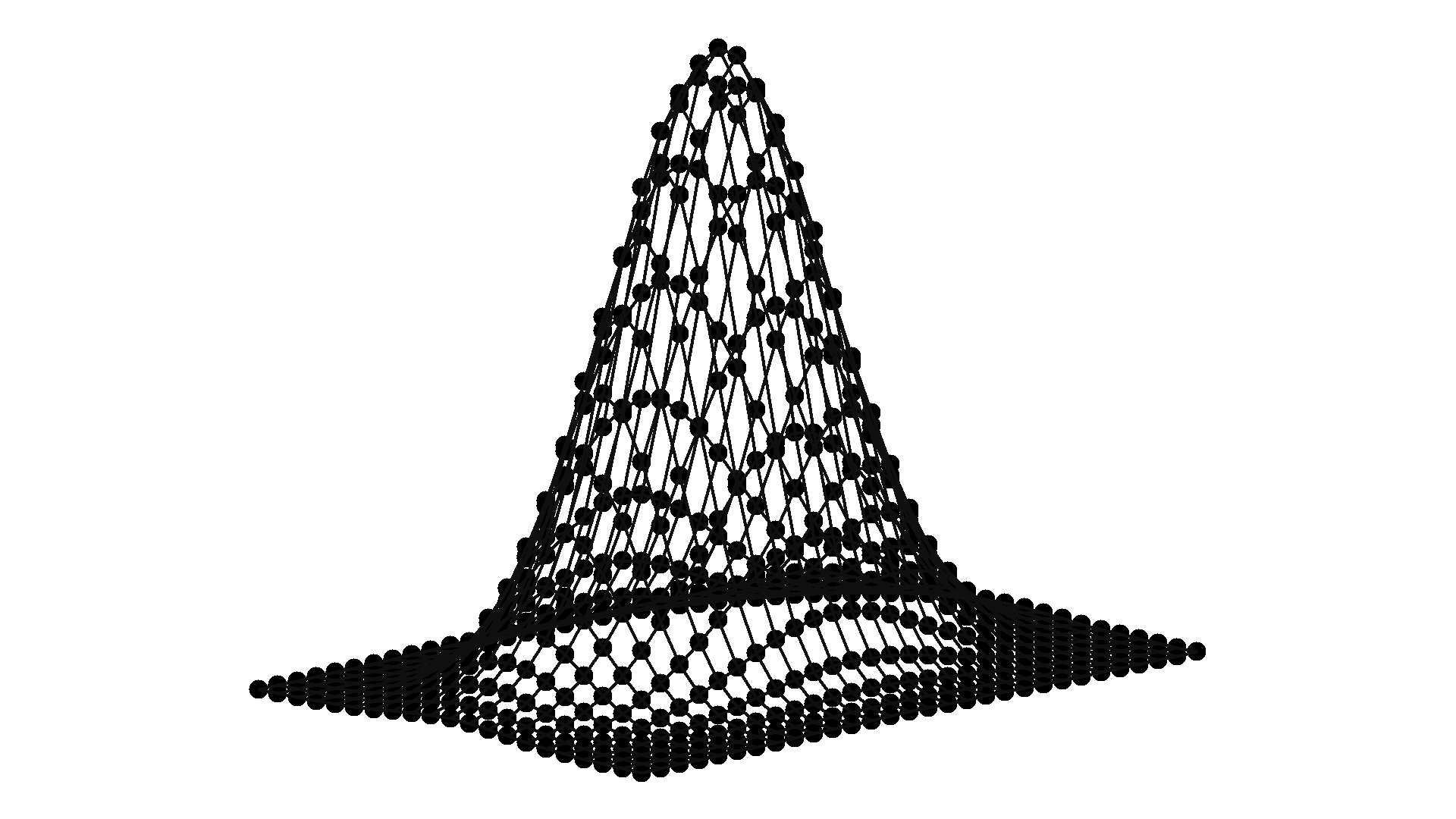### Example 4: Two-colored 3D-Plot

. graph3d x y z, colorscheme(cr)
[Try also . graph3d x y z x, colorscheme(cr)]
[and . graph3d x y z w, colorscheme(cr)]### Example 5: Two-colored 3D-Plot with changing markers

. graph3d x y z, colorscheme(cr) mark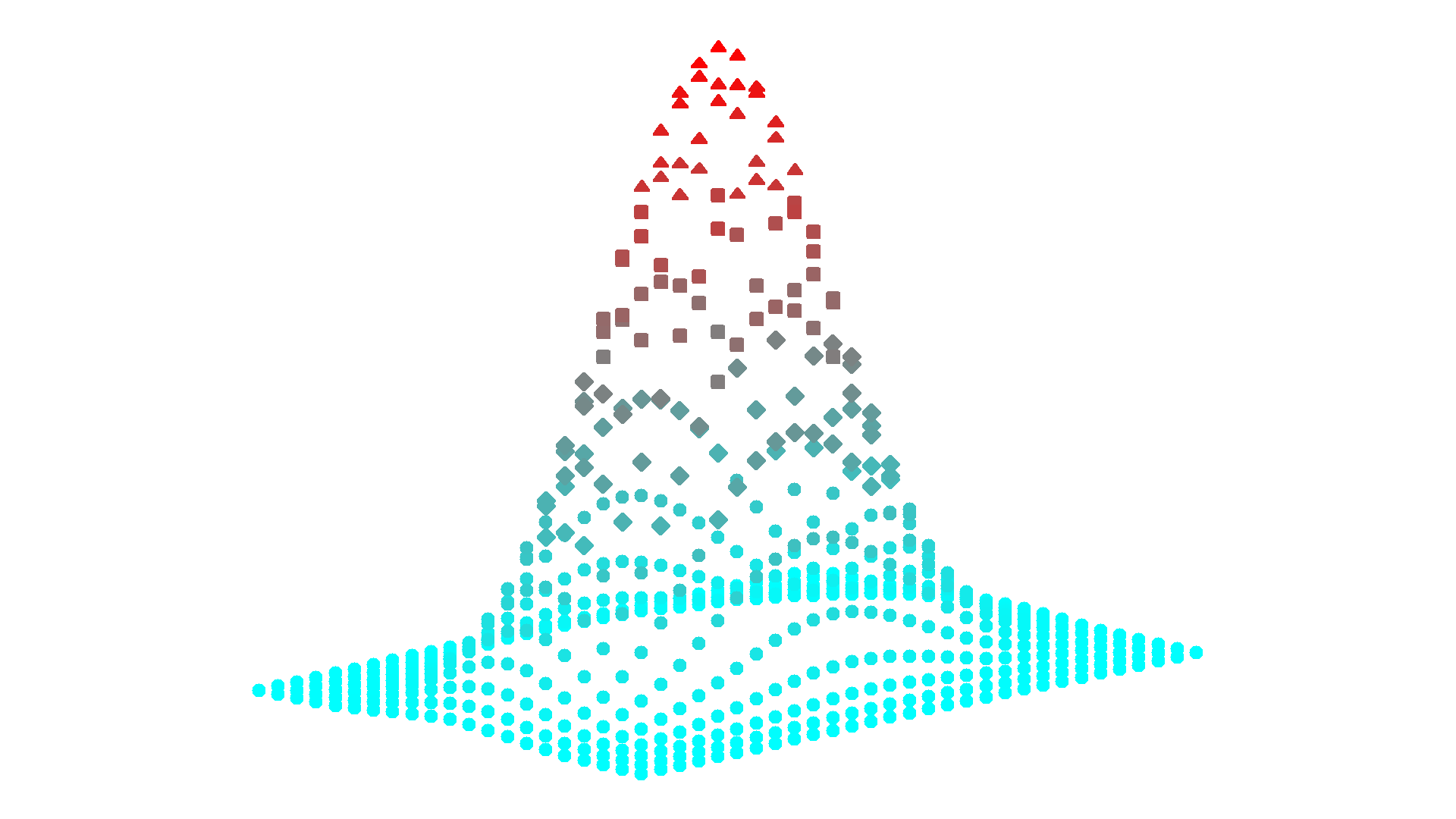### Example 6: Equidistantly two-colored 3D-Plot with changing markers

. graph3d x y z, colorscheme(cr) mark equi### Example 7: Five-colored 3D-Plot with changing markers

. graph3d x y z, colorscheme(bcgyr) mark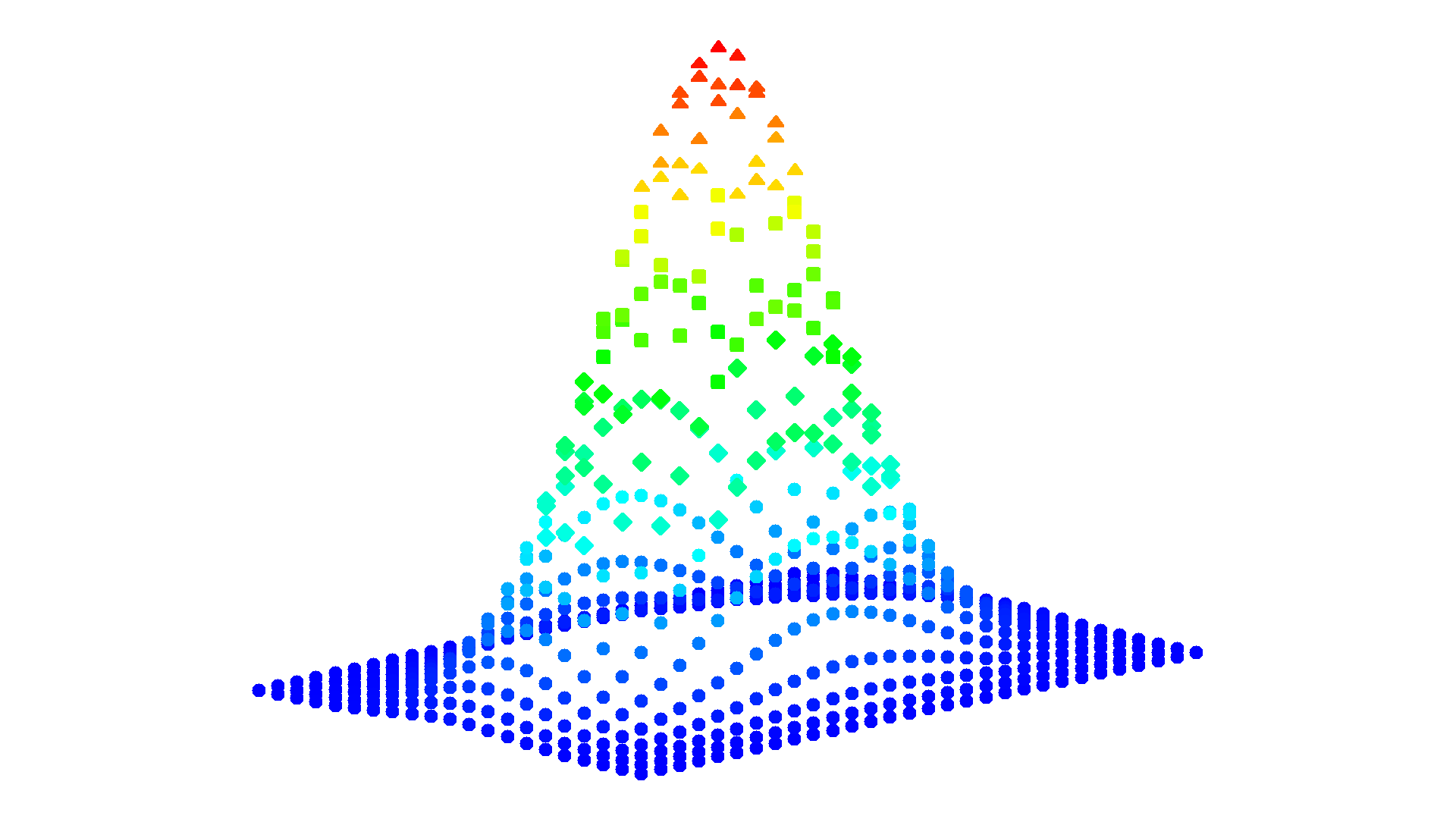### Example 8: Grey scale 3D-Plot

. graph3d x y z, colorscheme(fade)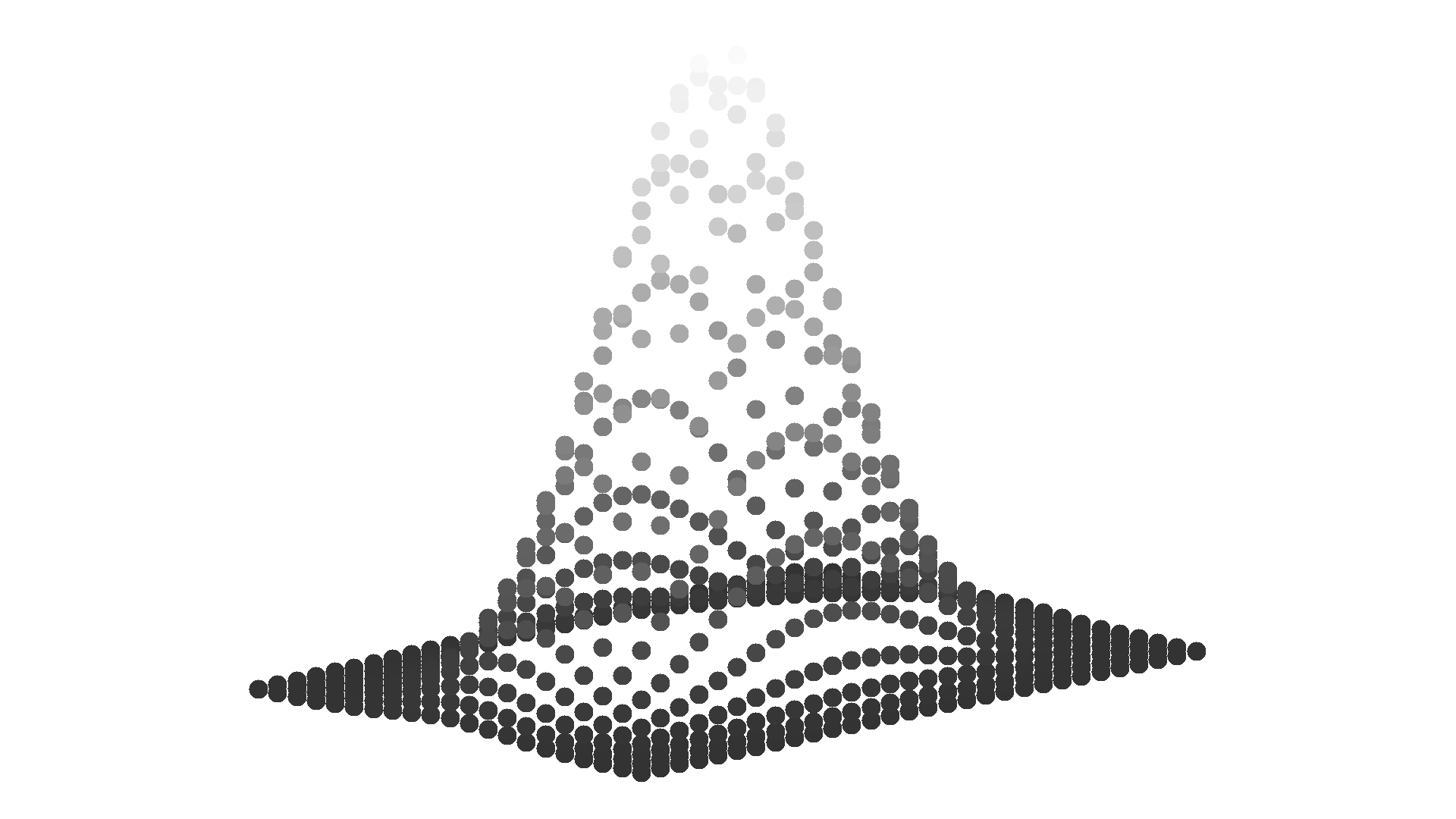### Example 9: Snowball 3D-Plot

. graph3d x y z, markeroptions(mfcolor(white) mlcolor(black) msize(3))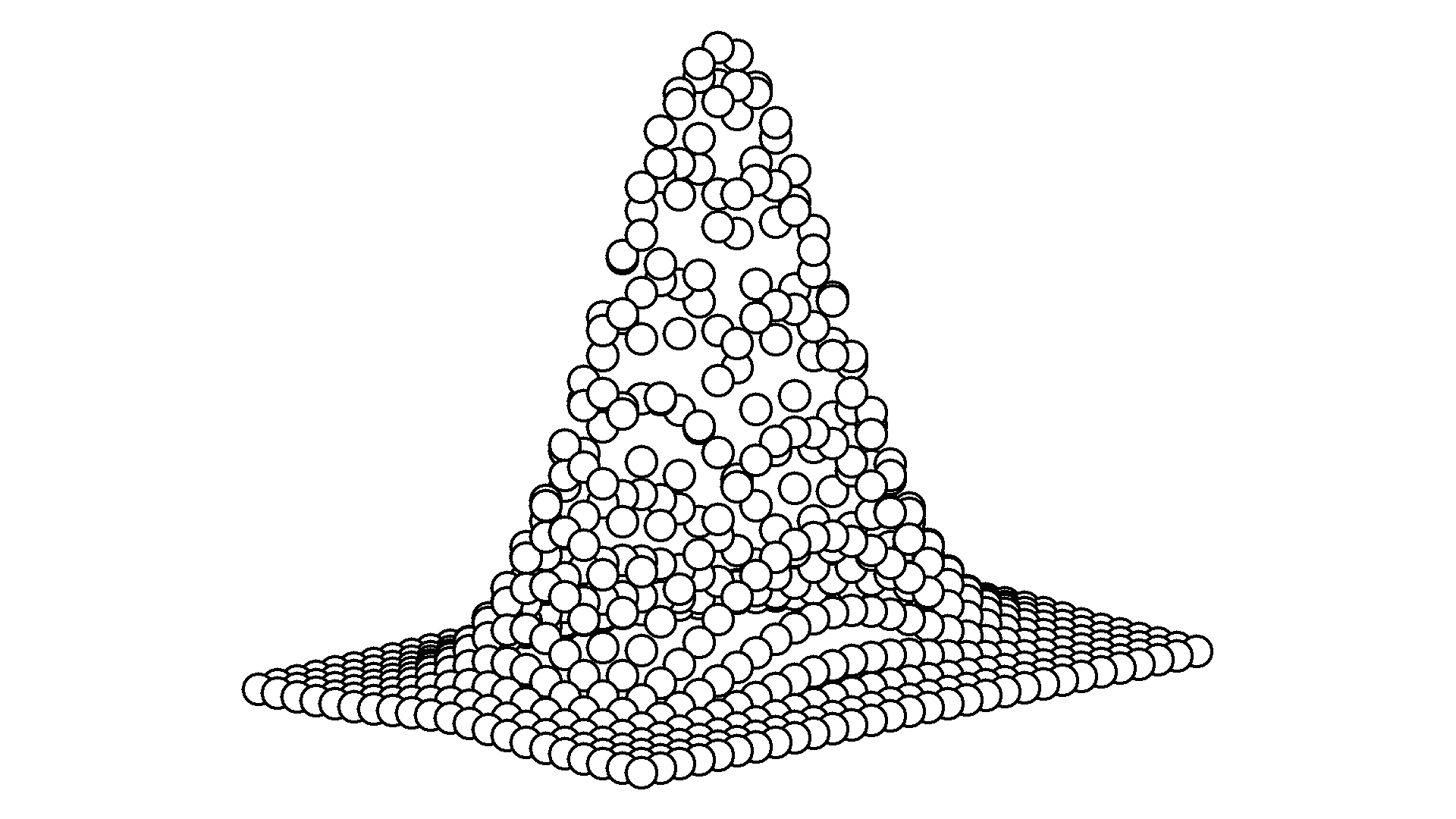### Example 10: Default 3D-Plot with outer grid-cuboid

. graph3d x y z, cuboid format("%03.0f")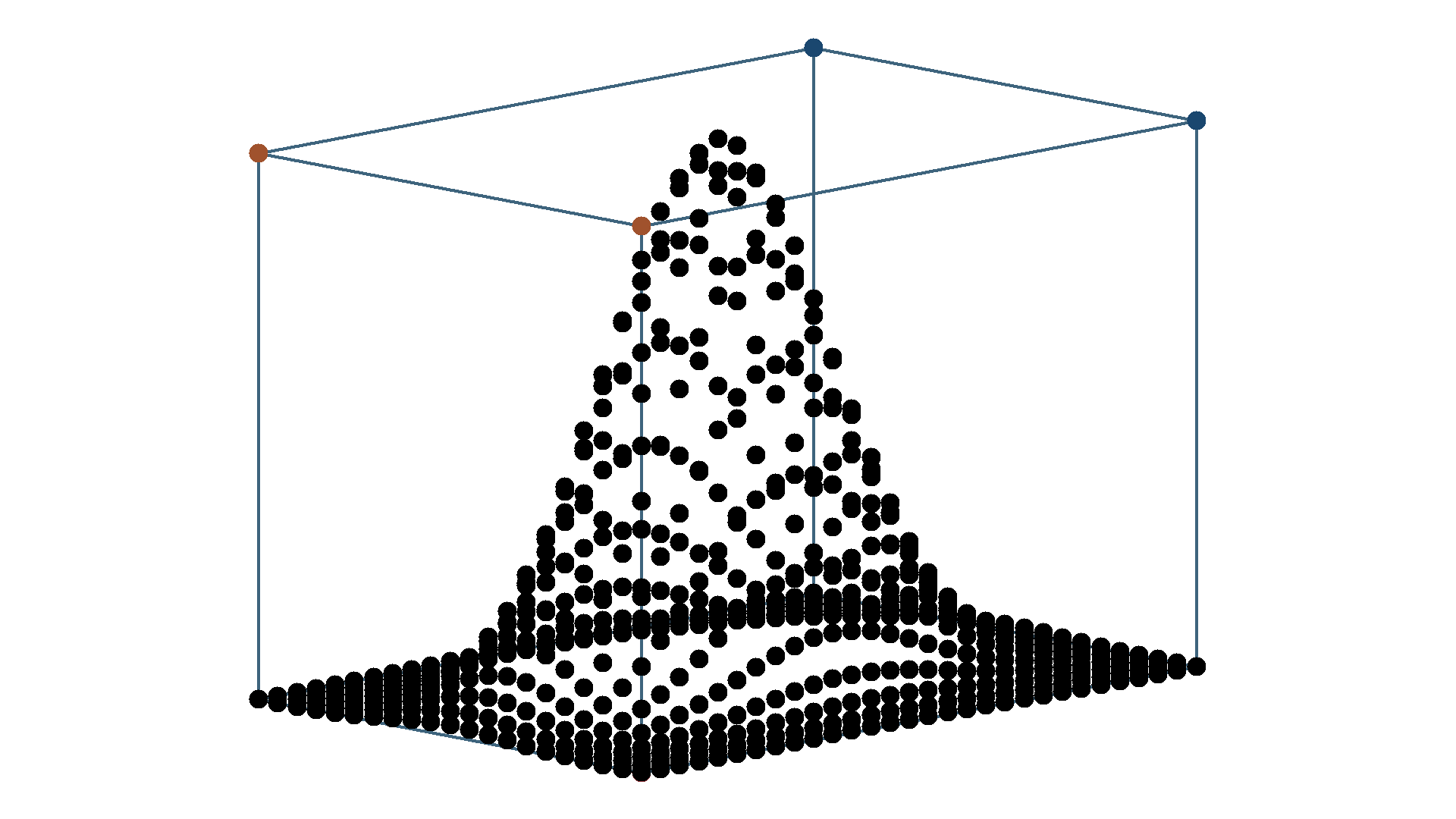### Example 11: Default 3D-Plot with outer and inner grid-cuboids

. graph3d x y z, cuboid innergrid format("%03.0f") blv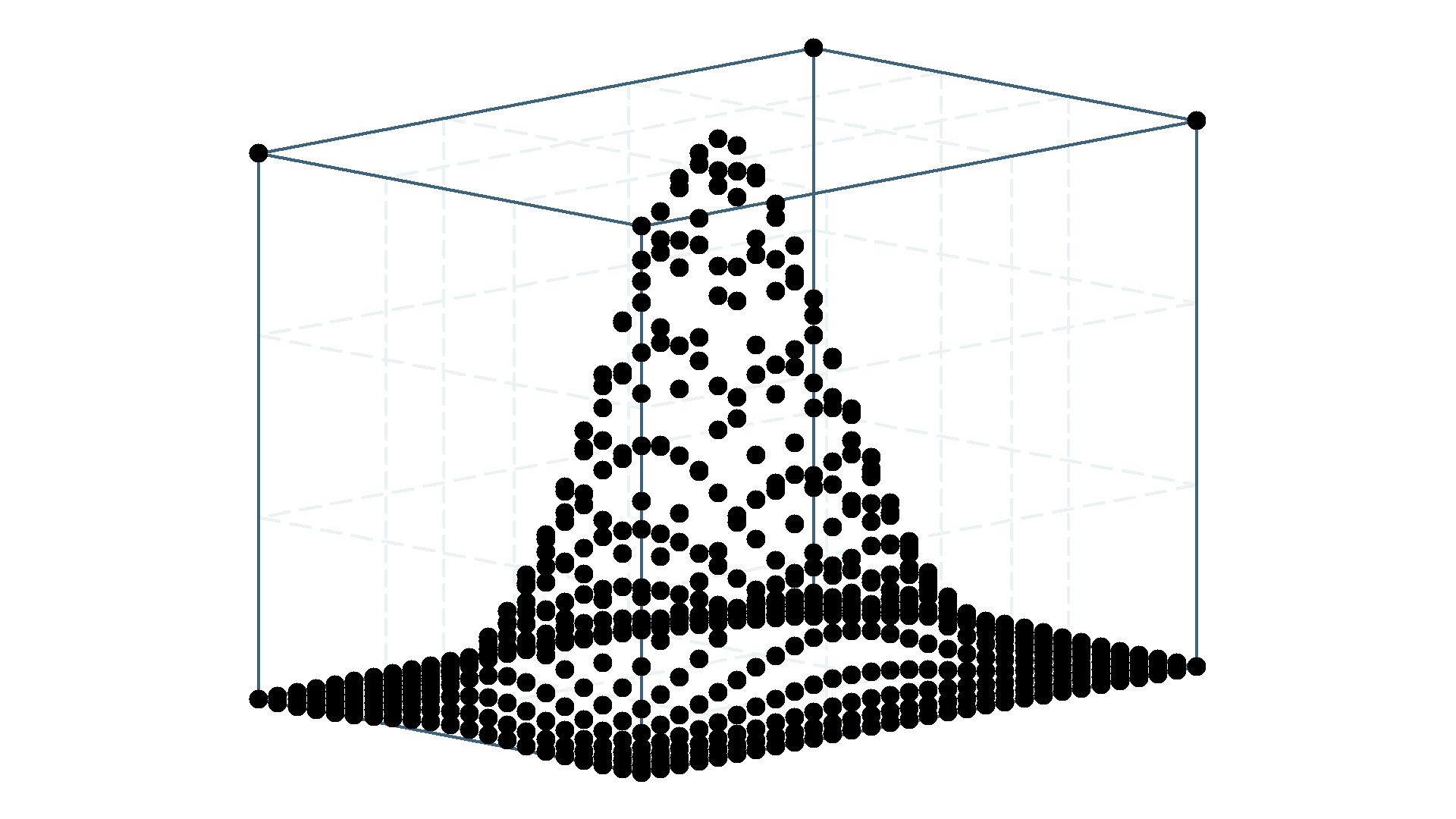### Example 12: Perspective 3D-Plot

. graph3d x y z, ycam(-4) zcam(-18) cuboid innergrid blv perspective colorscheme(bcgyr)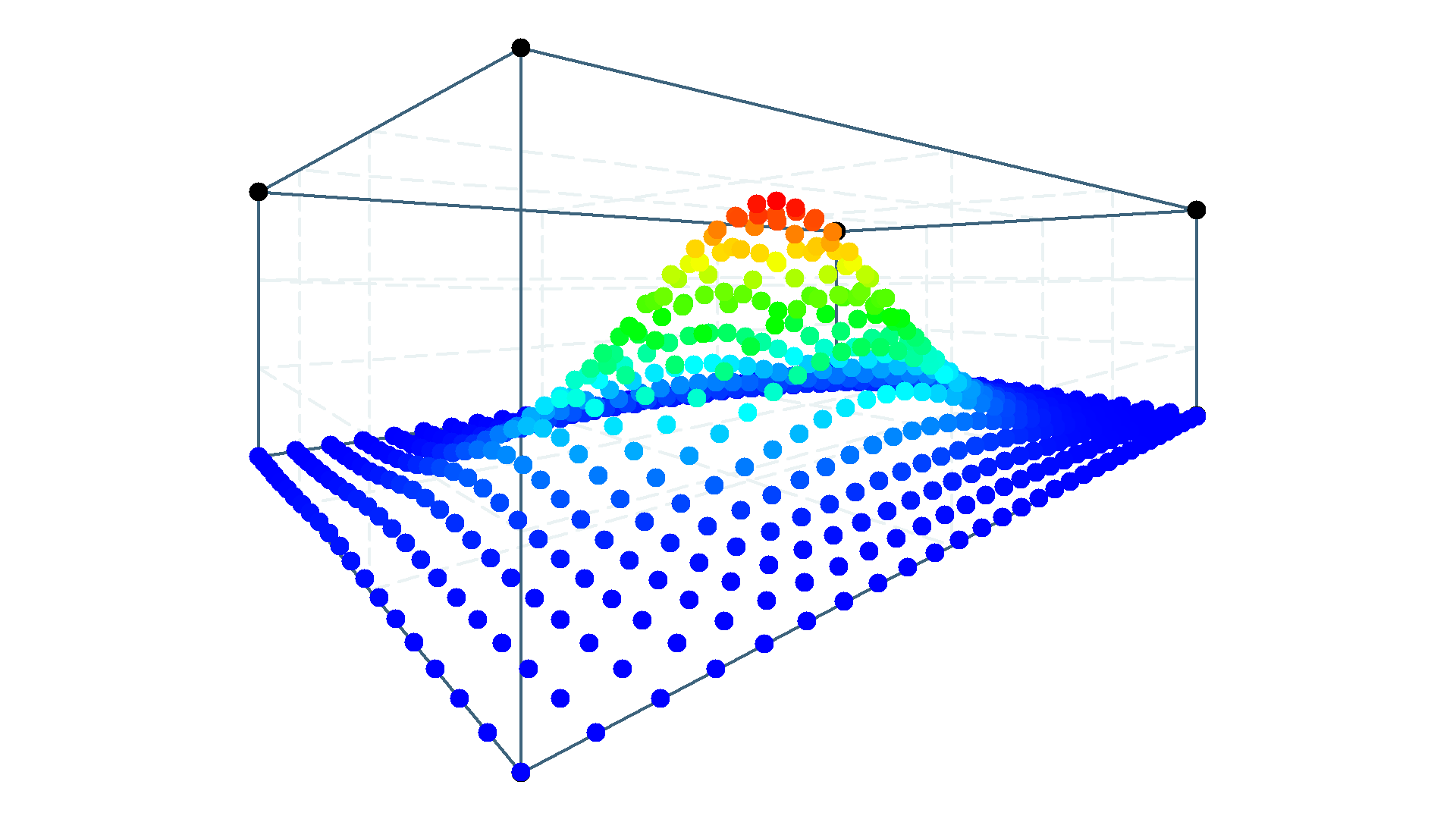### Example 13: Four-colored 2D-Plot

. graph3d x y z, xang(0) yang(0) zang(0) colorscheme(bcgyr)### Example 15: graph3d for margins plot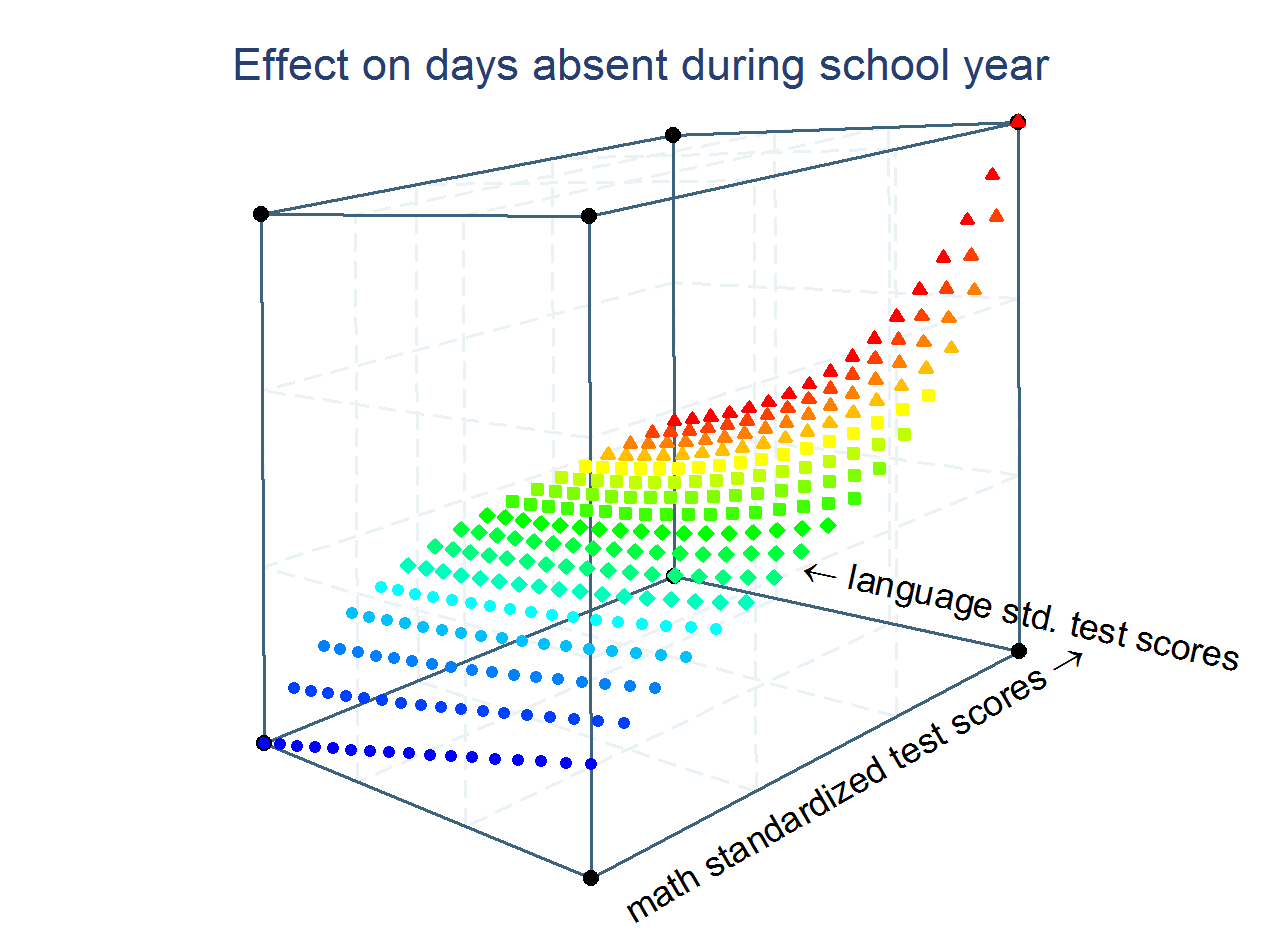### Example 16: Happy Valentine's Day